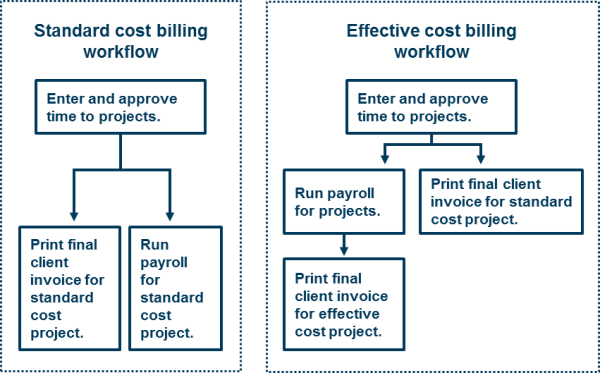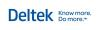You are here: Ajera Help (A-Z) > Accounting and industry concepts > About standard vs. effective cost

# About standard vs. effective cost

## What is standard cost?

In Ajera, standard cost is the expected cost of a salaried employee, not the actual cost (which is called effective cost).

Differences between standard and actual costs are called variances. With standard cost, the variance goes to the Payroll Variance account.

Ajera uses standard cost for costing and billing by default.

Here is an example of how to calculate the standard cost of a salaried employee:

• An employee's salary is \$1,000.
• The employee is paid weekly for a normal pay period of 40 hours, regardless of how many hours are actually worked.
• The standard hourly cost rate is \$25 (\$1,000/40 hours).
• All employee time entered to a project is assessed at \$25 per hour.
• If the employee worked less or more than 40 hours, the variance goes to the Payroll Variance account.

## What is effective cost?

In Ajera, effective cost is the actual cost of a salaried employee, not the expected cost.

With effective cost, Ajera corrects the variance between the standard cost and the actual cost when you run payroll.

Before you run payroll, the project and general ledger information reflects standard cost. When you run payroll, Ajera calculates the effective cost rate and replaces the standard cost in the project and general ledger information with effective cost.

If you process payroll in-house or with a payroll service, you can use effective cost for costing, billing, or both if a project requires it.

Here is an example of how to calculate the effective cost of a salaried employee:

• An employee's salary is \$1,000.
• The employee is paid weekly and worked 50 hours during the pay period.
• The effective hourly cost rate is \$20 (\$1,000/50 hours).
• All employee time entered to a project is assessed at \$25 per hour (\$1,000/40 hours) before you process payroll. After payroll, this rate is reassessed at the effective cost rate of \$20 per hour (\$1,000/50 hours).
 Note: If you are paying a salaried employee overtime in addition to regular salary, the overtime hours will be excluded from the effective cost calculation.

## How does Ajera handle standard cost and effective cost in billing?

You can bill some projects at effective cost and other projects at standard cost in Ajera, depending on the project requirements.

• For standard cost projects, you can invoice salaried time whenever you want.
• For effective cost projects, you can invoice salaried time if the billing rate tables are set to use effective cost only after running payroll, which is when Ajera calculates the effective cost rates. To learn more, see About effective cost.

## What are the different billing workflows?

Standard cost and effective cost billing require different workflows.© 2015 Deltek Inc. All rights reserved.